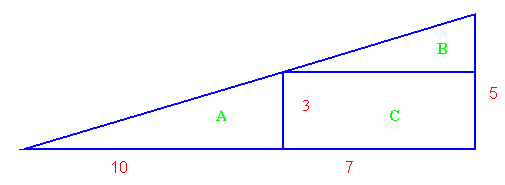#### You may also like### Kissing Triangles

Determine the total shaded area of the 'kissing triangles'.### Isosceles

Prove that a triangle with sides of length 5, 5 and 6 has the same area as a triangle with sides of length 5, 5 and 8. Find other pairs of non-congruent isosceles triangles which have equal areas.Four rods, two of length a and two of length b, are linked to form a kite. The linkage is moveable so that the angles change. What is the maximum area of the kite?

# Disappearing Square

##### Age 11 to 14 Challenge Level:

Do you know how to find the area of a triangle?

You can count the squares.

What happens if we turn the triangle on end? Play the video to see.

Try counting the number of units in the triangle now.

Do you have any interesting findings to report?

The area of a triangle can be found by multiplying half the base by the height. What is the area of this whole triangle?Now find the areas of the shapes marked A, B and C.

If you add these answers together, would they equal the same as the whole triangle? Try and see.

Do you have any interesting findings to report?